Chapter Notes: Statistics

# Statistics Chapter Notes - Mathematics (Maths) Class 9

## Introduction

Every day we come across a lot of information in the form of facts, numerical figures, tables, graphs, etc. as shown figures below. For example:

• Runs scored by a team,
• Profits made by a company,
• Temperatures recorded in a day of a city,
• Expenditures in various sectors by government,
• The weather forecast, election results, and so on.

These facts or figures, which are numerical or otherwise, collected with a definite purpose are called data.
Therefore, Data is a collection of facts, such as numbers, words, measurements, observations, etc.

Suppose, the following image shows the performance of the Indian Cricket team in tests in the last 2 years against major test playing nations.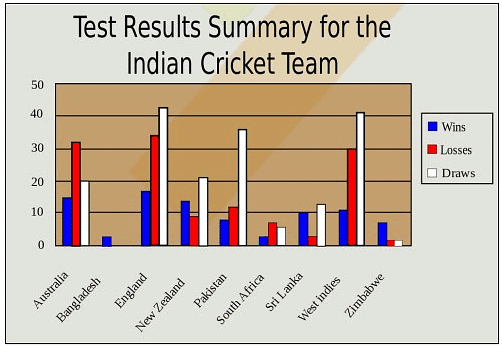Types of data based on the collection of facts
Qualitative data: It is descriptive data. For examples:

• Rajan is thin.
• Suman can run fast.
• The cake is orange in color.
• She has black hair.
• He is tall.

Quantitative data: It is numerical information. For examples:

• I updated my phone 6 times in a quarter
• She has 10 holidays in this year
• 500 people attended the seminar
• 54% of people prefer shopping online instead of going to the mall.

### Types of Quantitative data

Discrete data: It has a particular fixed numerical value that can be counted. For example, in the image below, there are 12 students in the class. Here, the number of students in the class is a discrete  data.Continuous data: It does not have a fixed value but a range of data. For example, in the figure below, the height of the 3 persons lie between 3 feet to 5 feet.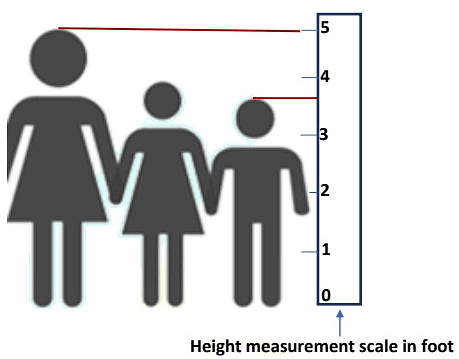### Collection of Data

Our world is becoming more and more information oriented. Every part of our life utilizes information in one form or another. So, it becomes essential for us to know how to extract meaningful information from such data. The extraction of meaningful information is studied in a branch of mathematics called Statistics.

This involves the study of the collection, analysis, interpretation, presentation, and organization of data. In other words, it is a mathematical discipline to collect, summarize data.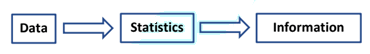Types of data on the basis of the collection of data
Primary data: It is the data that is collected by a researcher from first-hand sources, using methods like surveys, interviews, or experiments. For example, The following data is collected by a student for his/her thesis for the research project.

• Height of 10 students in your class.
• Number of absentees in each day in your class for a month.
• Number of members in the families of your classmates.
• Height of 10 plants in or around your home.

Secondary data: It is the data that has already been collected by someone, and then it is updated, tailored or modified for a specific purpose.
For example, in a school, the class-teachers of respective sections record attendance on a daily basis.
This data recorded by the class- teacher is an example of primary data. On a given day, the principal of the school asks for the attendance of all students of each section, to collate the total number of students present in the school on a given day. This data collected by the school principal is an example of secondary data.

### Presentation of data

After the collection of data, we need to arrange the data such that it is easily understood, meaningful and serves the required purpose.

Such an arrangement is called the presentation of data. The raw data can be arranged in any of the following ways:

• Serial order or alphabetical order
• Ascending order
• Descending order
• Frequency Distribution

When the raw data is arranged in ascending or descending order, then the data is called an array or arrayed data.
Suppose that the marks obtained by 10 students of class 9th in a mathematics test, out of 50 marks according to their roll numbers be: 39, 45, 33, 19, 21, 41, 21, 19, 40, 41.
The data in this form are called raw data or ungrouped data. The above raw data can be arranged according to in serial order (roll number) as follows: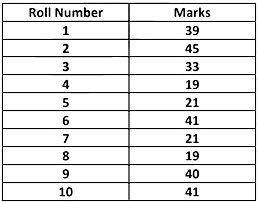Suppose, we want to find out who scored maximum or minimum marks in the test. The data in the given form does not give us a clear understanding of the performance of the students.
If we arrange the marks scored in ascending or descending order, it gives us a better understanding of the given data. Also, we can easily identify the minimum and maximum values in the data.
In ascending order, the data looks as follows:
19, 19, 21, 21, 33, 39, 40, 41, 41, 45. ⇒ Array or arrayed data.
In descending order, the data looks as follows:
45, 41, 41, 40, 39, 33, 21, 21, 19, 19. ⇒ Array or arrayed data.
From the above array or arrayed data, we can easily identify that the minimum marks are 19 and maximum marks are 45.

### Frequency distribution

• Suppose, 40 students appeared for a mathematics test. There is a possibility that the score of two or more students is the same. To find out how many students scored the same marks, we use a frequency distribution. If there is more than one instance like this, then finding that data may be time-consuming.
• So, when the number of observations is large, then arranging the data in serial order or ascending order or descending order can be quite time consuming and also it does not tell us much except the minimum and maximum of the given data.
• To minimize this effort and easily understand the data, we tabulate the data in a frequency distribution table.

Frequency distributions are of two types: Ungrouped frequency distribution table and Grouped frequency distribution table.
Let us consider a large data like the marks obtained (out of 100 marks) by 40 students of class 9th of a school.
50, 60, 70, 21, 19, 33, 39, 21, 92, 88, 80, 70, 72, 19, 40,
41, 92, 50, 50, 56, 60, 70, 60, 60, 88, 41, 45, 92, 88, 95,
70, 40, 39, 33, 19, 21, 41, 45, 70, 80.
If we arrange them in ascending order, it gives us a slightly better picture.
19, 19, 19, 21, 21, 21, 33, 33, 39, 39, 40, 40, 41, 41,
41, 45, 45, 50, 50, 50, 56, 60, 60, 60, 60, 70, 70, 70, 70,
70, 72, 80, 80, 88, 88, 88, 92, 92, 92, 95.
But, in the data arranged above, we cannot easily find how many students scored 41 marks or 60 marks. Again, we have to count that.
To make data easily understandable and clear, we can tabulate data of 40 students as shown below.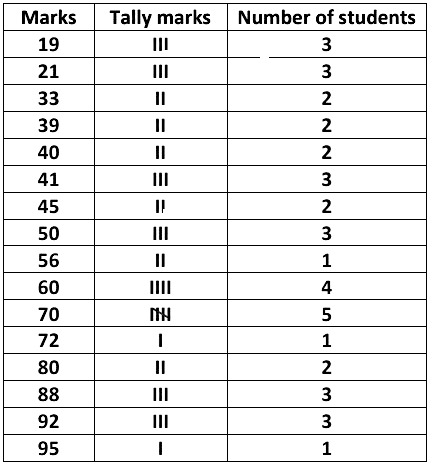• Marks are called variates and the number of students who secured a particular number of marks is called the frequency of the variate.
• The number of times an observation occurs in the given data is called the frequency of the observation.
• This way of presentation of data is known as frequency distribution or ungrouped frequency distribution table.

In the above table, we can observe how many students scored the same marks but we have recorded 19 such observations. In the even bigger data than this, we may have to draw much bigger tables which makes our work cumbersome and time-consuming. To overcome this limitation, we can represent that data in classes or groups as shown below: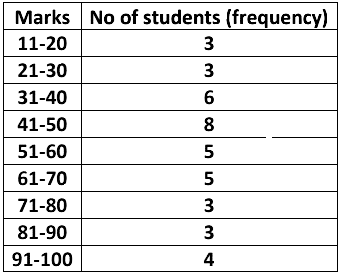In the table above, we have grouped the marks obtained by students in groups, which are called the classes or class intervals and their size is called the class-size or class width. Class 11-20 means the marks obtained between 11 and 20 including both 11 and 20. The number of observations falling in a particular class is called the frequency of that class or class frequency.

• Class size of the above data is 9. We find it by finding the difference between the upper limit and the lower limit of the class interval.
• For example, for the class interval 11-20, the smaller value 11 is the lower limit and the greater value 20 is the upper limit.
• Now, the class-size or class width for the above data = (20 – 11) = (30 – 21) = (40 – 31) = (50 – 41) = (60 – 51) = (70 – 61) = (80 – 71) = (90 – 81) = (100 – 91) = 9.
• This type of presentation of data is called a grouped frequency distribution table. Presenting data in this form simplifies and condenses data and enables us to observe certain important features at a glance.

We can prepare Grouped frequency distributions by two methods:
I. Inclusive Method
II. Exclusive Method

Inclusive Method: In this method, the classes are so formed that the upper limit of a class is included in that class. For example: In the class 11-20 of marks obtained by students, a student who has obtained 20 marks is included in this class.
Grouped frequency distribution table:1 is shown below which is arranged by an inclusive method.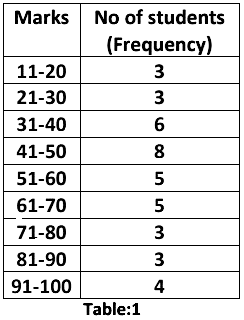Suppose, two new students are admitted in the class 9th whose marks are 20.5 and 30.5, but we cannot add them in the class intervals viz. 11-20 and 21-30 as these values are not included in any of these class intervals. To include marks 20.5 and 30.5, we use
Exclusive Method for preparing grouped frequency distribution. So, to include marks 20.5 and 30.5, we need intervals such that the upper limit of a class interval should be the same as the lower limit of the next class interval.

Exclusive Method: The class intervals are formed such that the upper limit of a class interval is the same as the lower limit of the next class interval. This method is called the exclusive method of classification.
Grouped frequency distribution table:2 is shown below which is arranged by an exclusive method.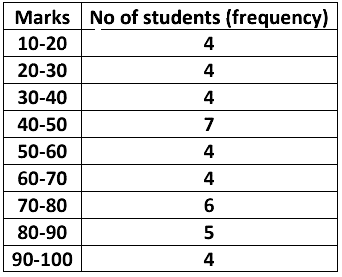In this method, the upper limit of a class is not included in the class.
For example, if a student scores 20 marks, then it is included in the class 20-30 but not in the class 10-20. So, any observation which is common to two class intervals, then it shall be considered in the higher class interval.
Example: Given below are the ages of 25 students of class 9th in a school. Prepare a ungrouped frequency distribution and grouped frequency distribution table.
15, 16, 16, 17, 17, 16, 15, 15, 16, 16, 17, 15, 16, 16,  14, 16, 15, 14, 16, 15, 14, 15, 16, 16, 15, 14, 15.

In the given data the observations are only 14, 15, 16 and 17. These ages are repeated multiple times. So, 14, 15, 16 and 17 are variates of data.
Frequency distribution of the ages of 25 students is given below.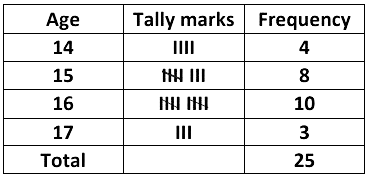For a grouped frequency distribution table, we decide class interval according to own convenience.
Grouped frequency distribution table including exclusive method of ages of 25 students are given below.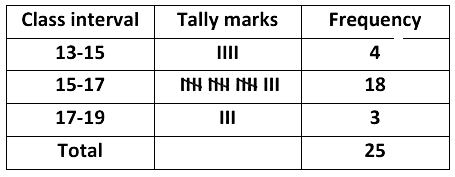### Graphical Representation of Data

A graphical representation is the visual display of data and its statistical results. It is more often and effective than presenting data in tabular form. Bar graphs, Histograms, and frequency polygons are different types of graphical representation, which depends on the nature of the data and the nature of statical results.

Bar Graphs: Bar graphs are the bars of uniform width that can be drawn horizontally or vertically with equal spacing between them and then the length of each bar represents the given number. Such a method of representing data is called a bar diagram or a bar graph.
For a clear representation of categorical data or any ungrouped discrete frequency observations, we generally use the bar graphs.

Example 1: Considering the modes of transport of 30 students of class 9th is given below:In order to draw the bar graph for the data above, we prepare the frequency table as given below.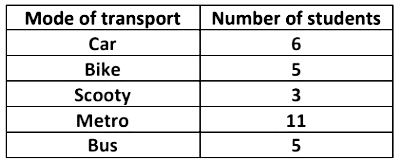Now, we can represent this data using a bar graph, by following the steps as shown below:

• First, we draw two axes viz. x–axis and y–axis. Then, we decide what each axis of the graph represents. By convention, the variates being measured goes on the horizontal (x–axis) and the frequency goes on the vertical (y–axis).
• Next, decide on a numeric scale for the frequency axis. This axis represents the frequency in each category by its height. It must start at zero and include the largest frequency.
• Having decided on a range for the frequency axis we need to decide on a suitable number scale to label this axis. This should have sensible values, for example, 0, 1, 2, . . . , or 0, 10, 20 . . . , or other such values as to make sense given the data.
• Draw the axes and label them appropriately.
• Draw a bar for each category. When drawing the bars it is essential to ensure the following:
• the width of each bar is the same
• the bars are separated from each other by equally sized gaps.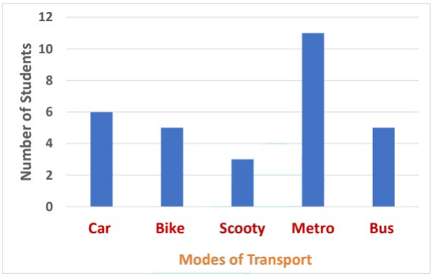Using this bar graph, we can easily identify the most popular mode of transport is the metro. Bar graphs provide a simple method of quickly spotting patterns within a discrete data set.

Histograms
Histogram was first introduced by Karl Pearson in 1891. Bar charts have their limitations; like they cannot be used to represent continuous data. When dealing with continuous random variables different kinds of graphs are used. This type of graph is called a histogram.
At first sight, a histogram looks similar to bar charts. However, there are two critical differences:

• The horizontal (x-axis) is a continuous scale. As a result of this, there are no gaps between the bars (unless there are no observations within a class interval).
• The height of the rectangle is only proportional to the frequency of the class if the class intervals are all equal. With histograms, it is the area of the rectangle that is proportional to their frequency.

Example 2: Consider the weights of 20 students of a class 9th as  given below: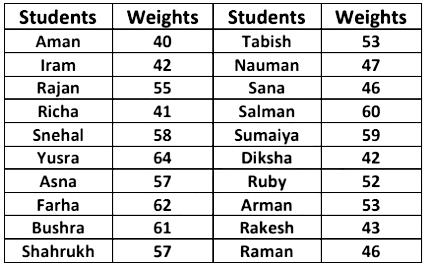Now, arranging the data in ascending order.
40, 41, 42, 42, 43, 46, 46, 47, 52, 53, 53, 55, 57, 57, 58, 59, 60, 61, 62, 64.
In order to draw the histogram for the data above, we prepare the frequency table as given below.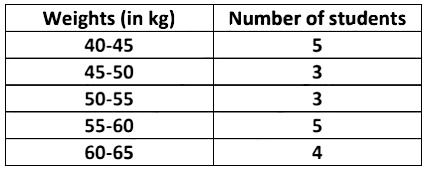We can represent this information using histogram, by following steps as shown below:

• Find the maximum frequency and draw the vertical (y–axis) from zero to this value.
• The range of the horizontal (x–axis) needs to include a full range of the class intervals from the frequency table.
• Draw a bar for each group in your frequency table. These should be the same width and touch each other (unless there are no data in one particular class).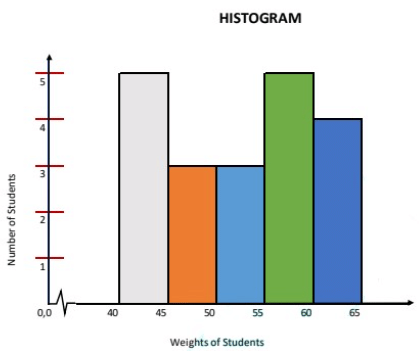Frequency Polygon
It is a natural extension of the histogram. In frequency polygon rather than drawing bars, each class is represented by one point and these are joined together by straight lines. We draw frequency polygons in a similar way of drawing a histogram.

Example 3: Consider the weights of 20 students of a class 9th as given below: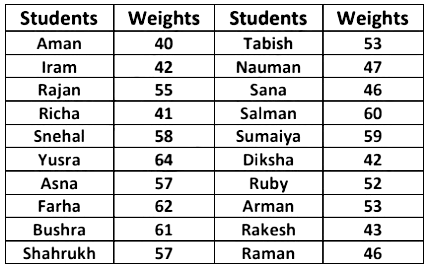Now, arranging the data in ascending order.
40, 41, 42, 42, 43, 46, 46, 47, 52, 53, 53, 55, 57, 57, 58, 59, 60, 61, 62, 64.
In order to draw the frequency polygon for the data above, we prepare the frequency table as given below.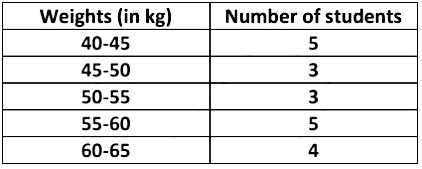We can then present this information as a frequency polygon, by following the process of the steps shown below:

• Prepare a frequency table.
• Find the maximum frequency and draw the vertical (y–axis) from zero to this value.
• The range of the horizontal (x–axis) needs to include all class intervals from the frequency table.
• Draw bars for each class interval in the frequency table. These bars should be of the same width and are adjacent to each other (unless there are no data in one particular class)
• Connect the midpoints of the top side of each bar by a dotted line as shown below.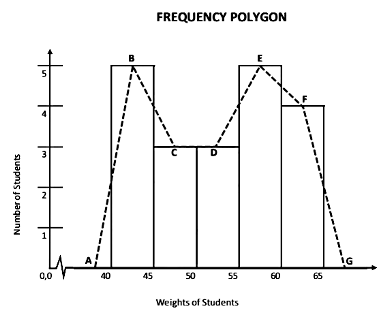Frequency polygons can also be drawn independently without drawing histograms. For this, we require the mid-points of the class-interval. These mid-points of the class intervals are called class marks.
Classmark = Upper Limit + Lower Limit  / 2

### Measures of Central Tendency

A measure of central tendency represents the center point of data. These measures indicate where most values in a distribution lie and are also referred to as the central location of a distribution.

There are three main measures of central tendency: the mean, the median, and the mode.
Mean (Average): It is calculated by dividing the sum of all observations in data by the number of observations. So, if we have n observations in a data set and they have observations x1, x2, ...,xn, the sample mean, usually denoted by x̅ (read as x bar), is: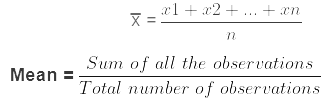This formula is usually written in a slightly different manner using the Greek capital letter, Σ, read as "sigma", which means sum of: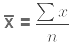Where x = x1 + x2 + ........ + xn.

Example 1: Find the mean of the marks obtained by 20 students of class 9th of a school :
20, 30, 30, 10, 40, 45, 30, 20, 25, 45, 10, 25, 35, 45, 40, 20, 30, 25, 20,10.

Suppose that x1= 20, x2 = 30, x3 = 30, x4 = 10, x5 = 40, x6 = 45, x7 = 30, x8 = 20, x9 = 25, x10 = 45, x11 = 10, x12 = 25, x13 = 35, x14 = 45, x15 = 40, x16 = 20, x17 = 30, x18 = 25, x19 = 20 & x20 = 10.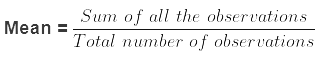Therefore,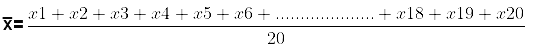Where,
= 20 + 30 + 30 + 10 + 40 + 45 + 30
+ 20 + 25 + 45 + 10 + 25 + 35
+ 45 + 40 + 20 + 30 + 25 + 20
+ 10
= 555
Therefore, x̅  = 555/20 = 27. 75.
So, the mean of the marks obtained by 20 students of class 9th = 27.75.

Median: The median is the middle observation for a set of data that has been arranged in either ascending or descending order. Median is that observation that splits the arranged data into two halves.
For an even and odd number of observations in ungrouped data, we have different approaches to find the median.

• When the number of observations (n) is odd, the median is the value of the (n + 1/2)th observation. For example, if n = 11, the value of the (n/2+1)th is equal to 6th observation, which is the median as shown in the figure below.• When the number of observations (n) is even, the median is the mean of the (n/2)th and the (n/2 +1)th observations.
If n = 10 then the mean of the (10/2)th and the(10/2 + 1)th observations, i.e. the mean of the values of the 5th and 6th observations is the median, as shown in the figure below.Example 2: The heights (in cm) of 11 students of a class 9th are as follows:
155, 160, 140, 130, 145, 135, 150, 152, 160, 142, 144.

First of all, we arrange the data in ascending order, as follows:
130, 135, 140, 142, 144, 145, 150, 152, 155, 160, 160.
Since the number of students is 11, an odd number, we find out the median by finding the height of the (n + 1/2)th = (11 + 1/2)th = the 6th students, which is 145 cm. [where n is the number of students]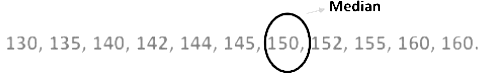Mode: It is defined as the most frequently occurring observations in data. That is, an observation with the maximum frequency is called the mode.Example 4: The heights (in cm) of 12 students of a class 9th are as follows:
155, 160, 140, 130, 145, 135, 150, 152, 160, 142, 144, 160.

We can arrange the given data in ascending order:
130, 135, 140, 142, 144, 145, 150, 152, 155, 160, 160, 160.
Here 160 cm occurs most frequently, i.e. three times. So, the mode is 160 cm.

Example 5: The heights (in cm) of 12 students of a class 9th are as follows:
150, 160, 140, 144, 143, 153, 153, 155, 160, 160, 155, 155.

We can arrange the given data in ascending order:
140, 143, 144, 150, 153, 153, 155, 155, 155, 160, 160, 160.
Here, 155 cm and 160 cm all occur most frequently (three times).
So, the mode is 155 cm and 160 cm.

### Summary of Statistics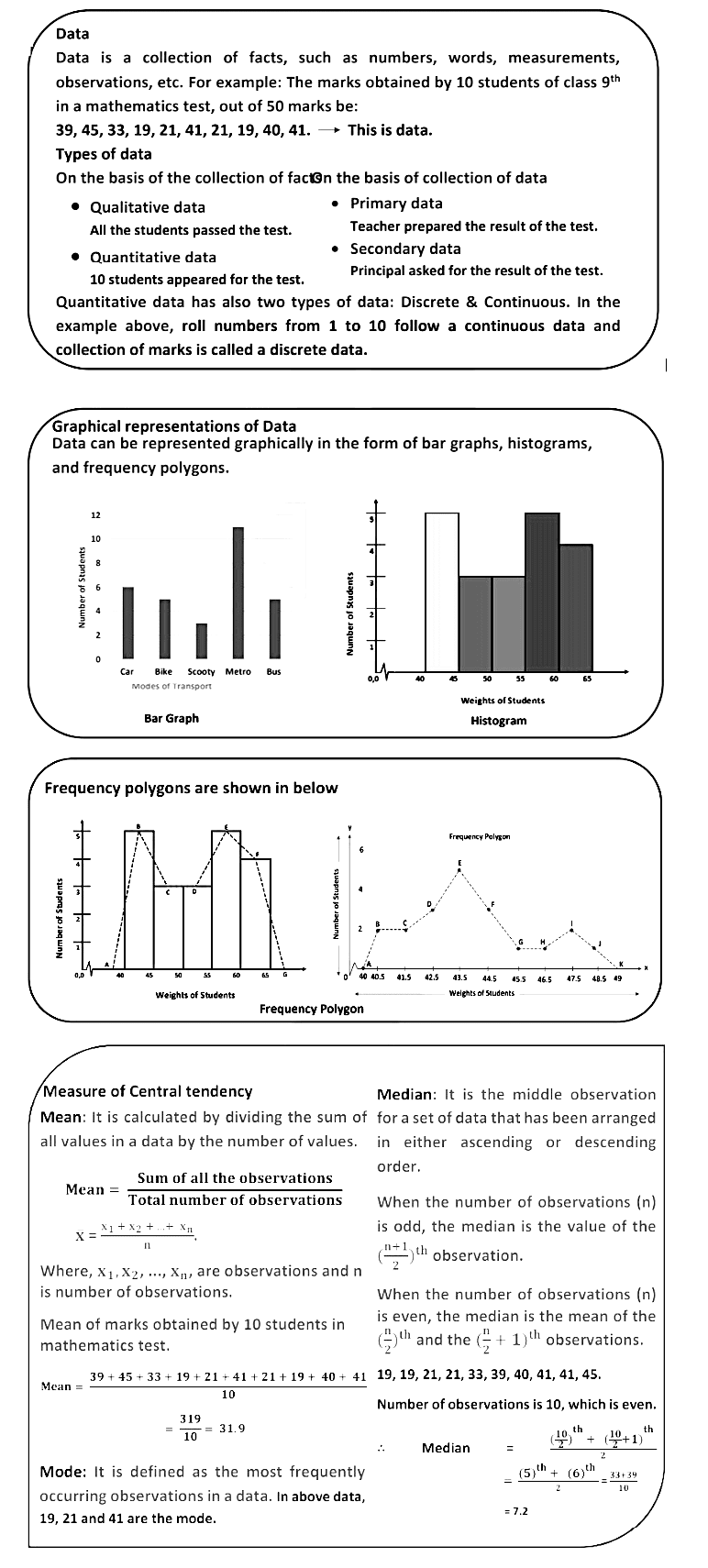The document Statistics Chapter Notes | Mathematics (Maths) Class 9 is a part of the Class 9 Course Mathematics (Maths) Class 9.
All you need of Class 9 at this link: Class 9

## FAQs on Statistics Chapter Notes - Mathematics (Maths) Class 9

 1. What is statistics and why is it important?Ans. Statistics is a branch of mathematics that involves collecting, analyzing, interpreting, and presenting data. It helps us make sense of the information around us and make informed decisions. Statistics plays a crucial role in various fields such as economics, business, medicine, social sciences, and more.
 2. What are the different types of data in statistics?Ans. In statistics, data can be categorized into four types: nominal, ordinal, interval, and ratio. Nominal data represents categories or labels, such as gender or ethnicity. Ordinal data represents a ranking or order, such as rating scales. Interval data has equal intervals between values, such as temperature measurements. Ratio data has a meaningful zero point, such as weight or height.
 3. How do you calculate the mean, median, and mode in statistics?Ans. The mean is calculated by adding up all the values in a dataset and dividing it by the number of values. The median is the middle value in a dataset when arranged in ascending or descending order. The mode is the value that appears most frequently in a dataset.
 4. What is the difference between population and sample in statistics?Ans. In statistics, a population refers to the entire group of individuals or objects of interest. It is often too large to study entirely. A sample, on the other hand, is a smaller subset of the population that is selected to represent the whole. Data is collected from the sample and then used to make inferences about the population.
 5. How do you interpret a standard deviation in statistics?Ans. Standard deviation measures the spread or variability of a dataset. A smaller standard deviation indicates that the data points are close to the mean, while a larger standard deviation indicates that the data points are more spread out. It helps assess the consistency or variability of the data.

## Mathematics (Maths) Class 9

62 videos|426 docs|102 tests

## Mathematics (Maths) Class 9

62 videos|426 docs|102 tests
Signup to see your scores go up within 7 days! Learn & Practice with 1000+ FREE Notes, Videos & Tests.
10M+ students study on EduRev
Track your progress, build streaks, highlight & save important lessons and more!(Scan QR code)
Related Searches

,

,

,

,

,

,

,

,

,

,

,

,

,

,

,

,

,

,

,

,

,

;